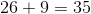# ISEE Lower Level Quantitative : How to find the missing part of a list

## Example Questions

← Previous 1

### Example Question #67 : Data Analysis And Probability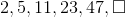What is the next number in the series?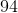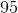Explanation:

First, determine what the pattern is in the series. The pattern here is to multiply the previous number by 2 and then add 1. Therefore, multiply 47 by 2 (which is 94), and then add 1. The result is 95.

### Example Question #68 : Data Analysis And Probability

What is the missing value of w in this sequence?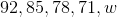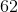Explanation:

In this sequence, every subsequent number is 7 less than the preceding number. Given that the number that precedes w is 71, the value of w is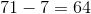. Therefore, 64 is the correct answer.

### Example Question #69 : Data Analysis And Probability

What is the value of n in the sequence below?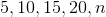Explanation:

The numbers increase by 5. Given that the number before n is 20, the value of n is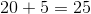.

### Example Question #3 : Nth Term Of An Arithmetic Sequence

What is the next term in the following sequence?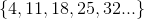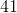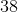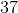Explanation:

What is the next term in the following sequence?This is an arithmetic sequence with a common difference of. To find the next term in an arithmetic sequence, add the common difference to the previously listed term: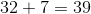### Example Question #71 : Data Analysis And Probability

Find the missing number in the sequence: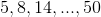Explanation:

In this sequence, the difference between each number is double the difference between the number that came prior to it in the sequence. For example,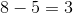and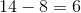. Thus, the missing number must be greater thanby a difference ofand therefore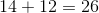### Example Question #72 : Data Analysis And Probability

What is the missing number in the following list: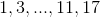Explanation:

In this sequence of numbers, there is an important pattern to recognize. In this sequence each number is a prime number, however the sequence skips every other prime number. Thus, the sequence begins with the prime numberand then skips the prime number(which is the only even prime number) and goes to. Fromthe next prime number is, however since the pattern in this sequence skips every other prime number--the missing number is.

### Example Question #73 : Data Analysis And Probability

Find the missing number in this set: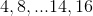Explanation:

In this sequence of numbers, there is an important pattern to recognize. Each number in this sequence is a composite number--however, the sequence skips every other composite number. Thus, the next composite number afteris--which is skipped because of the pattern, making the correct answer.

### Example Question #74 : Data Analysis And Probability

Find the next number in the list: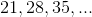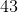Explanation:

In this sequence of numbers, there is an important pattern to recognize. Each number is greater than the number prior to it by a margin of. In other words, each number in this list is a multiple of. Thus,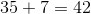### Example Question #75 : Data Analysis And Probability

Find the missing number: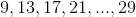Explanation:

In this sequence of numbers, there is an important pattern to recognize. Each number is greater than the number prior to it by a margin of. Thus, the correct answer is, because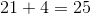### Example Question #76 : Data Analysis And Probability

Find the missing number in the list below: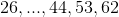In this sequence of numbers, there is an important pattern to recognize. Each number is greater than the number prior to it by a margin of 9. Thus, the correct answer isbecause